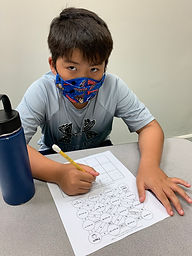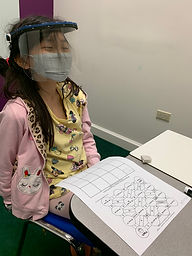## Ms. Kayla

### Target 1​

###### Lesson Type:

Review

Number Operation

:

Integer Composition

Decompose numbers to determine the relationship between two whole numbers.

###### 1:

Identify the greatest common factor (GCF) of two whole numbers.

###### 2:

Compute the greatest common factor (GCF) using prime factorization.

6th

###### Vocabulary:

Factor, Multiple, Composite and Prime numbers, Greatest Common Factor (GCF)

Activities:

Students had to identify the Greatest Common Factor (GCF) to navigate through a maze.### Home Exploration

###### Guiding Questions:## Absent Students:

### Target 2

:

###### 1:

Use ratios of corresponding sides within a figure to create similar figures or determine whether two figures are similar.

###### 2:

Understand that when figures are scaled, the side lengths are proportional.

###### 3:

Use a given center point to enlarge a figure.

7th

###### Vocabulary:

Dilation, Scale drawing, Ratio, X-axis, Y-axis, Enlarge, Proportion, Double

Activities:

Students started with transformation of an image to a bigger scale to introduce the concept of dilation.

Students then were to dilate another image on a coordinate grid with the ratio 1:2.### Home Exploration

###### Guiding Questions:### Target 3

:

###### Vocabulary:

Activities:### Home Exploration